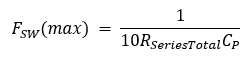cancel
Showing results for
Did you mean:

# Constant “10” in the Fsw(max) equation - KBA234212Community Manager

## Constant “10” in the Fsw(max) equation - KBA234212

Version: **

The maximum sense clock frequency [Fsw(max)] is calculated as follows:The selected sense clock frequency should be such that the sensor will charge and discharge completely in each sense clock period. The equation for the maximum sense clock frequency comes from the characteristics of the RC circuit.

In an RC circuit, it takes about 5 RC until the capacitor is completely discharged. Because the discharge is performed in half the time of one cycle, the time of one cycle is T = 2 * 5RC = 10RC; the frequency is calculated as 1 / T; therefore, it is 1/10 RC.

See AN85951 – PSoC™ 4 and PSoC™ 6 MCU CAPSENSE™ design guide for more information.

Version history
Revision #:
1 of 1
Last update:
‎Nov 22, 2021 09:47 PM
Updated by:Contributors